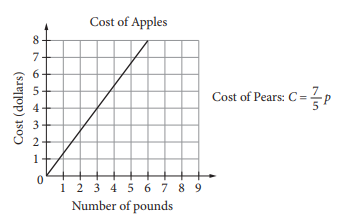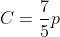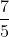Scan QR code or get instant email to install app

Question:

# The graph above shows the cost, in dollars, of apples as a function of the number of pounds of apples purchased at a particular grocery store. The equation above defines the cost C, in dollars, for p pounds of pears at the same store. Which of the following statements accurately compares the cost per pound of apples and the cost per pound of pears at this store?A Apples cost approximately \$0.07 less per pound than pears do.
explanation

1212The cost per pound of apples can be determined by the slope of the graph as about 1.33 per pound. The cost per pound of pears can be determined by the slope of the line defined by the equation. The slope of the line defined by C is, so the cost per pound of pears is 1.40. Therefore, the apples cost approximately 0.07 less per pound than pears do.